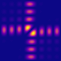QPMS Electromagnetic multiple scattering library and toolkit.
beyn.h
Go to the documentation of this file.
1
4 #ifndef BEYN_H
5 #define BEYN_H
6
7 #include <stddef.h>
8 #include <complex.h>
9
11
12 typedef int (*beyn_function_M_t)(complex double *target_M, size_t m, complex double z, void *params);
13
15
16 typedef int (*beyn_function_M_inv_Vhat_t)(complex double *target_M_inv_Vhat, size_t m, size_t l,
17  const complex double *Vhat, complex double z, void *params);
18
20 typedef struct beyn_contour_t {
21  size_t n;
23
29  complex double centre;
31  _Bool (*inside_test)(struct beyn_contour_t *, complex double z);
32  complex double z_dz[];
34
36
37 beyn_contour_t *beyn_contour_ellipse(complex double centre, double halfax_re, double halfax_im, size_t npoints);
38
39
40 typedef enum {
41  BEYN_CONTOUR_HALFELLIPSE_RE_PLUS = 3,
42  BEYN_CONTOUR_HALFELLIPSE_RE_MINUS = 1,
43  BEYN_CONTOUR_HALFELLIPSE_IM_PLUS = 0,
44  BEYN_CONTOUR_HALFELLIPSE_IM_MINUS = 2,
45 } beyn_contour_halfellipse_orientation;
46
47
49
50 beyn_contour_t *beyn_contour_halfellipse(complex double centre, double halfax_re, double halfax_im, size_t npoints,
51  beyn_contour_halfellipse_orientation or);
52
54 beyn_contour_t *beyn_contour_kidney(complex double centre, double halfax_re, double halfax_im,
55  double rounding,
56  size_t n, beyn_contour_halfellipse_orientation or);
57
58
60 typedef struct beyn_result_t {
61  size_t neig;
62  size_t vlen;
63  complex double *eigval;
64  complex double *eigval_err;
65  double *residuals;
66  complex double *eigvec; // Rows are the eigenvectors
67  double *ranktest_SV;
68
70
71 void beyn_result_free(beyn_result_t *result);
72
75  size_t m,
76  size_t l,
78  beyn_function_M_inv_Vhat_t M_inv_Vhat,
79  void *params,
80  const beyn_contour_t *contour,
81  double rank_tol,
82  size_t rank_min_sel,
83  double res_tol
84  );
85
86 #endif // BEYN_H
int(* beyn_function_M_inv_Vhat_t)(complex double *target_M_inv_Vhat, size_t m, size_t l, const complex double *Vhat, complex double z, void *params)
(optional) User-supplied function that, given , calculates .
Definition: beyn.h:16
int(* beyn_function_M_t)(complex double *target_M, size_t m, complex double z, void *params)
User-supplied function that provides the (row-major) m × m matrix M(z) whose "roots" are to be found.
Definition: beyn.h:12
beyn_result_t * beyn_solve(size_t m, size_t l, beyn_function_M_t M, beyn_function_M_inv_Vhat_t M_inv_Vhat, void *params, const beyn_contour_t *contour, double rank_tol, size_t rank_min_sel, double res_tol)
Solve a non-linear eigenproblem using Beyn's algorithm.
Definition: beyn.c:506
beyn_contour_t * beyn_contour_kidney(complex double centre, double halfax_re, double halfax_im, double rounding, size_t n, beyn_contour_halfellipse_orientation or)
Similar to halfellipse but with rounded corners.
Definition: beyn.c:161
struct beyn_contour_t beyn_contour_t
Complex plane integration contour structure.
beyn_contour_t * beyn_contour_halfellipse(complex double centre, double halfax_re, double halfax_im, size_t npoints, beyn_contour_halfellipse_orientation or)
Complex plane "half-elliptic" integration contour with axes parallel to the real, imaginary axes.
Definition: beyn.c:111
struct beyn_result_t beyn_result_t
Beyn algorithm result structure (pure C array version).
beyn_contour_t * beyn_contour_ellipse(complex double centre, double halfax_re, double halfax_im, size_t npoints)
Complex plane elliptic integration contour with axes parallel to the real, imaginary axes.
Definition: beyn.c:61
A VSWF representation element of the qpms_l_t l
< The O(3) element in the quaternion representation.
Definition: quaternions.h:214
Complex plane integration contour structure.
Definition: beyn.h:20
complex double centre
"Centre" of the contour.
Definition: beyn.h:29
_Bool(* inside_test)(struct beyn_contour_t *, complex double z)
Function testing that a point z lies inside the contour (optional).
Definition: beyn.h:31
complex double z_dz[]
Pairs of contour points and derivatives in that points.
Definition: beyn.h:32
size_t n
Definition: beyn.h:21
Beyn algorithm result structure (pure C array version).
Definition: beyn.h:60
size_t neig
Number of eigenvalues found.
Definition: beyn.h:61
size_t vlen
Vector space dimension (also the leading dimension of eigvec).
Definition: beyn.h:62